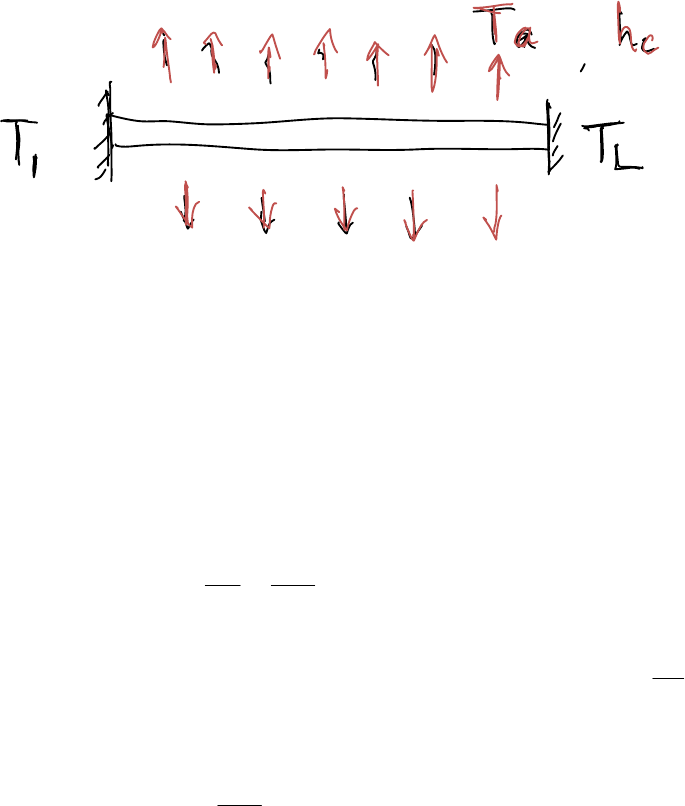Class Notes (1,100,000)
CA (620,000)
U of A (10,000)
CH E (100)
Lecture 7

# CH E374 Lecture Notes - Lecture 7: Stabilisation Force In Bosnia And Herzegovina, Secant Method, Rhode Island Route 2

Department
Chemical Engineering
Course Code
CH E374
Professor
Joe Mmbaga
Lecture
7

This preview shows pages 1-2. to view the full 7 pages of the document.1
Lecture Notes CHE 374 Computational Methods in Engineering
MODULE 7: Ordinary differential equations – Boundary value problems (BVP)
Basics
Figure 1
Consider a rod whose ends are maintained at constant temperatures, T1 and TL as shown
in Figure 1. Remember the heating rod problem. The rod loses heat to the environment
which is at a temperature Ta. (ambient temperature). The rate of heat loss by convection
from the sides of the rod depends on the heat transfer coefficient, hc, and the ambient
temperature, Ta. The partial differential equation governing temperature distribution as
a function of x and t is
2
2()
TT
hc Ta T
tx
∂∂
=+ −
∂∂ (7.1)
.
Suppose we are only interested in the steady-state solution. At steady-state 0
T
t
=
. Then
the PDE turns into an ODE:
2
2()0
dT hc Ta T
dx +−=
(7.2)
The two boundary conditions of this second-order ODE are 1
(0)Tx T== and
()
L
Tx L T==.
Note that the conditions are given at two different locations (x=0 and x=L). This is
called a boundary value problem. The solution methods for initial value problem
ODE’s (Module 6) can not directly deal with boundary value problems. In initial value

Only pages 1-2 are available for preview. Some parts have been intentionally blurred.2
problems the state of the system is fully known at one point (e.g. at t=0). From there on
we can march forward (e.g. with a Runge-Kutta method) to determine the solution at
any moment in time.
In boundary value problems, the state of the system is not fully known at one point and
we have no point to start our march from.
There are two solution approaches to boundary value problems, namely: The shooting
Method and Finite difference Method
Shooting Method
Suppose we can guess the boundary conditions required at one of the domains. We can
then solve this problem by using the initial value methods discussed in initial value
problem (module 6). If our guessed value gives the correct value at the end of the
domain, we have arrived, otherwise we have to guess again, and again until
convergence.
Finite difference Methods
Think again of the steady-state heating rod problem: we replace the function
(
)
Tx with
the temperature at a set of discrete points i
T (discretization) and transform the ODE and
the boundary conditions into a set of algebraic equations that we can solve with the
methods from Module 2 (non-linear equations) or Module 3 (linear equations) .
We explain these two methods in the following subsections:
The Shooting method
For (systems of) linear ODE’s the shooting method does not need iteration. As an
example we take a system of two linear ODE’s:
() () ()
() () ()
1112 23
241526
dy gx ygx ygx
dx
dy gx ygx ygx
dx
=+ +
=+ +
(7.3)
with boundary conditions
() ()
11,22,
and
s
f
ya y yb y==.